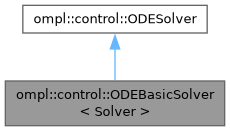ompl::control::ODEBasicSolver< Solver > Class Template Reference

Basic solver for ordinary differential equations of the type q' = f(q, u), where q is the current state of the system and u is a control applied to the system. StateType defines the container object describing the state of the system. Solver is the numerical integration method used to solve the equations. The default is a fourth order Runge-Kutta method. This class wraps around the simple stepper concept from boost::numeric::odeint. More...

#include <ompl/control/ODESolver.h>

Inheritance diagram for ompl::control::ODEBasicSolver< Solver >:[legend]

## Public Member Functions

ODEBasicSolver (const SpaceInformationPtr &si, const ODESolver::ODE &ode, double intStep=1e-2)
Parameterized constructor. Takes a reference to the SpaceInformation, an ODE to solve, and an optional integration step size - default is 0.01.Public Member Functions inherited from ompl::control::ODESolver
ODESolver (SpaceInformationPtr si, ODE ode, double intStep)
Parameterized constructor. Takes a reference to SpaceInformation, an ODE to solve, and the integration step size.

virtual ~ODESolver ()=default
Destructor.

void setODE (const ODE &ode)
Set the ODE to solve.

double getIntegrationStepSize () const
Return the size of a single numerical integration step.

void setIntegrationStepSize (double intStep)
Set the size of a single numerical integration step.

const SpaceInformationPtrgetSpaceInformation () const
Get the current instance of the space information.

## Protected Member Functions

void solve (StateType &state, const Control *control, double duration) const override
Solve the ODE using boost::numeric::odeint.Public Types inherited from ompl::control::ODESolver
using StateType = std::vector< double >
Portable data type for the state values.

using ODE = std::function< void(const StateType &, const Control *, StateType &)>
Callback function that defines the ODE. Accepts the current state, input control, and output state.

using PostPropagationEvent = std::function< void(const base::State *, const Control *, double, base::State *)>
Callback function to perform an event at the end of numerical integration. This functionality is optional.Static Public Member Functions inherited from ompl::control::ODESolver
static StatePropagatorPtr getStatePropagator (ODESolverPtr solver, const PostPropagationEvent &postEvent=nullptr)
Retrieve a StatePropagator object that solves a system of ordinary differential equations defined by an ODESolver. An optional PostPropagationEvent can also be specified as a callback after numerical integration is finished for further operations on the resulting state.Protected Attributes inherited from ompl::control::ODESolver
const SpaceInformationPtr si_
The SpaceInformation that this ODESolver operates in.

ODE ode_
Definition of the ODE to find solutions for.

double intStep_
The size of the numerical integration step. Should be small to minimize error.

## Detailed Description

### template<class Solver = odeint::runge_kutta4<ODESolver::StateType>> class ompl::control::ODEBasicSolver< Solver >

Basic solver for ordinary differential equations of the type q' = f(q, u), where q is the current state of the system and u is a control applied to the system. StateType defines the container object describing the state of the system. Solver is the numerical integration method used to solve the equations. The default is a fourth order Runge-Kutta method. This class wraps around the simple stepper concept from boost::numeric::odeint.

Definition at line 263 of file ODESolver.h.

The documentation for this class was generated from the following file: